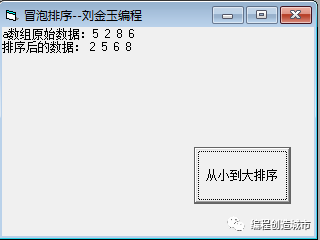# VB语言中如何实现冒泡排序法text1中的文本是5

text2中的文本是2

if val(text1.text)>val(text2.text) then

print text2.text  ’这里是小的值

else

print text1.text  '这里是大的值

end if

a(0)=5

a(1)=2

a(2)=8

a(3)=6

基准：以第一个数字作为基准。假设第一个数字是最小值，去跟后面所有的数进行比较。

第一轮：2  5  8   6

第二轮：2  5  8   6

第三轮：2  5  8   6

基准：以第二个数字作为基础。

第一轮：2  5  8   6

第二轮：2  5  8   6

基准：以第三个数字作为基准。

第一轮：2  5  6  8

'开始趟数

for i=0 to 2 step 1

’开始轮数

for j=1 to 3-i  step 1

if a(i)>a(j+i) then '实现交互

t=a(i)

a(i)=a(j+i)

a(j+i)=t

end if

next j

next i

``Option Explicit``Dim a(3) As Integer``Private Sub Command1_Click()``a(0) = 5``a(1) = 2``a(2) = 8``a(3) = 6``Dim i%, j%, t%``'开始趟数``  For i = 0 To 2 Step 1``    '开始轮数``    For j = 1 To 3 - i Step 1``       If a(i) > a(j + i) Then '实现交互``          t = a(i)``          a(i) = a(j + i)``          a(j + i) = t``       End If``    Next j``    ``  Next i``  ``  For i = 0 To 3 Step 1``    Print a(i)``  Next i``End Sub``